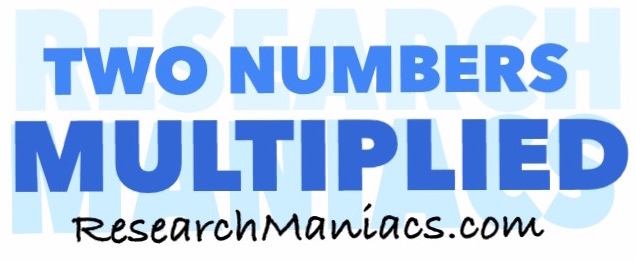What two numbers multiply to 116?What 2 numbers do you multiply to get 116? In other words, what number can you multiply with another number to get 116?

The formula to solve this would be:

X x Y = 116

There are at least two combinations of two numbers that you can multiply together to get 116.

For your convenience, we have made a list of all the combinations of two numbers multiplied by each other that will make 116:

1 x 116 = 116
2 x 58 = 116
4 x 29 = 116
29 x 4 = 116
58 x 2 = 116
116 x 1 = 116

Two Numbers Multiplied
Enter another number below to see which combinations of two numbers multiplied will equal that number.

What two numbers multiply to 117
Go here for the next number on our list.